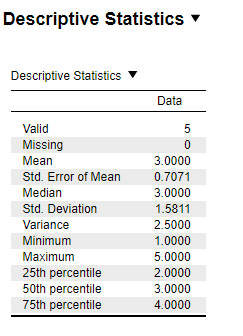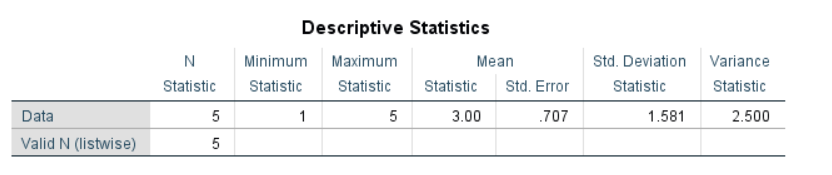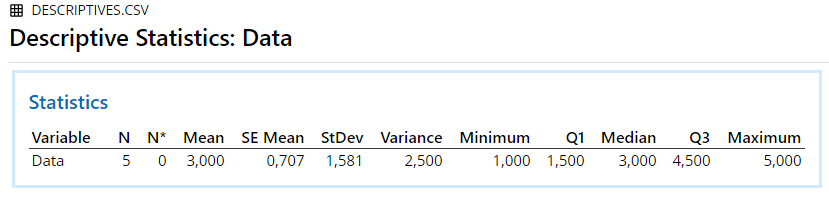# Chapter 2 Descriptives

## 2.1 Descriptives

An example from Kerlinger (1969, pp. 93-95):

Table 2.1: Data for Descriptives
Data
1
2
3
4
5

### 2.1.1 Results Overview

Table 2.2: Result Overview Descriptives
By Hand JASP SPSS SAS Minitab R
Mean 3.0 3.000 3.000 3.000 3.000 3.000
Variance 2.5 2.500 2.500 2.500 2.500 2.500
Median NA 3.000 3.000 3.000 3.000 3.000
Standard Deviation NA 1.580 1.580 1.580 1.580 1.580
SE(Mean) NA 0.707 0.707 0.707 0.707 0.707

### 2.1.2 By Hand

Calculations by hand can be found in Kerlinger (1969, pp. 93-95).

Result:

Mean = 3

Variance = 2.5

Note: Kerlinger calculated the population variance, however as all statistical software computes the sample variance, the formula was adapted accordingly to be divided by N-1.

### 2.1.3 JASPFigure 2.1: JASP Output for Descriptives

### 2.1.4 SPSS

DATASET ACTIVATE DataSet1.
DESCRIPTIVES VARIABLES=Data
/STATISTICS=MEAN STDDEV VARIANCE MIN MAX SEMEAN.Figure 2.2: SPSS Output for Descriptives

### 2.1.5 SAS

PROC MEANS DATA=work.Desc Mean STDDEV Median STDERR Var;
VAR Data;
RUN;Figure 2.3: SAS Output for Descriptives

### 2.1.6 MinitabFigure 2.4: Minitab Output for Descriptives

### 2.1.7 R

mean(desc.data2$Data) ##  3 sd(desc.data2$Data)
##  1.581139
var(desc.data2$Data) ##  2.5 median(desc.data2$Data)
##  3
se <- function(x) sqrt(var(x)/length(x))
se(desc.data2\$Data)
##  0.7071068

### 2.1.8 Remarks

All differences in results between the software and hand calculation are due to rounding.

### 2.1.9 References

Kerlinger, F. N. (1969). Foundations of behavioral research. New York, US: Holt, Rinehart and Winston, Inc.